# Catch up on the latest AI articles# A Block-based CNN Architecture Search Method Using Genetic Algorithms

3 main points
✔️ Designing CNN architecture requires a lot of domain knowledge and computational resources
✔️ Proposed an automatic block-based exploration of CNN architectures while saving computational resources
✔️ Comparing models explored by the proposed method with models designed by humans by hand and models explored by recent architectural automatic search algorithms in terms of error rate and required computational resources.

(Submitted on 21 July 2021)

The images used in this article are from the paper, the introductory slides, or were created based on them.

## first of all

CNN is a very successful model in the field of AI. However, its design is not easy. This is because the architecture of a CNN has a significant impact on its performance, and exploring its architecture requires a lot of domain knowledge and computational resources. A method to search the architecture of a neural network without requiring domain knowledge is called Neural Architecture Search (NAS), which has been studied extensively in recent years. NAS requires a large number of computational resources, and solving this problem has become a major topic of recent NAS research.

In this paper, to save computational resources, we explore architectures in blocks, which are a group of CNN structures to some extent. In addition, most of the NAS methods use reinforcement learning to search the architecture, but to save computational resources, we use our original genetic algorithm to search the architecture.

In our experiments, we compare three types of models: models explored by the proposed method, models designed by hand by humans, and models explored by recent architectural automatic search algorithms. In the comparison, we do not simply compare the error rates, but also compare the computational resources spent to search the model and the size (number of parameters) of the model.

## Related work

In this study genetic algorithm is used. The basic flow of the genetic algorithm is as follows

1. Randomly generate N individuals in the current generation
2. Calculate the degree of adaptation (performance) of each individual in the current generation, respectively, with the evaluation function
3. Perform any of the following three actions with a certain probability and store the result in the next generation
1. Two individuals are selected and mated
2. Select one individual to mutate.
3. Select one of the individuals and copy it as it is.
4. Repeat the above operation until the number of individuals in the next generation is N.
5. When the number of next generations reaches N, all the contents of the next generation are transferred to the current generation.
6. Repeat the operation after 2 for the maximum number of generations, and output the individual with the highest fitness in the last generation as the solution.

In this study, we use a unique method of mutation and a unique method of crossover.

## The proposed method (SaMuNet)

We now describe the details of "A self-adaptive mutation network (SaMuNet)" proposed in this study.

### The basic flow of SaMuNet

The basic flow of SaMuNet is similar to that of a basic genetic algorithm, as follows.

1. Randomly generate N individuals in the current generation
2. Calculate the degree of adaptation by the evaluation function
3. Select Parent in Tournament Selection
4. Generate offspring by crossover and mutation
5. Repeat 3~4 until there are N children.
6. Update the mutation probability of this generation (Self-adaptive mutation)
7. Make this generation your parents' generation.
8. Repeat 2~7 up to the maximum number of generations
9. Decode the final solution and get the CNN

### Encoding Strategy

In genetic algorithms, each individual is represented by a gene. The operation of converting the target to be searched into genes is called encoding, and the method of encoding is very important.

First, to represent the architecture of the CNN, we treat some chunks as blocks. For example, a ResNet block (RB) might look like this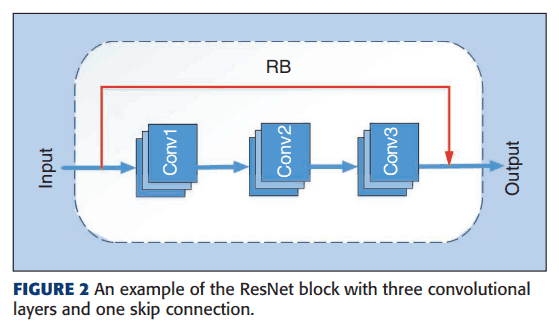Another example is the DenseNet block (DB), which is a block that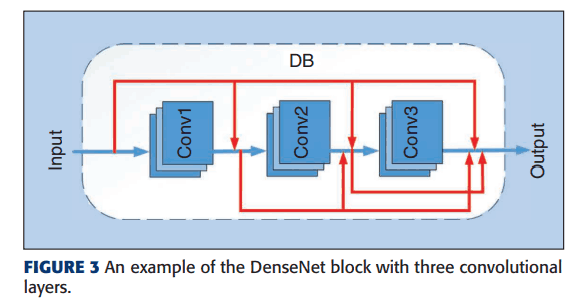A combination of these blocks to some extent is called a unit and is treated as a basic unit. One of the units, RU, will look like the following.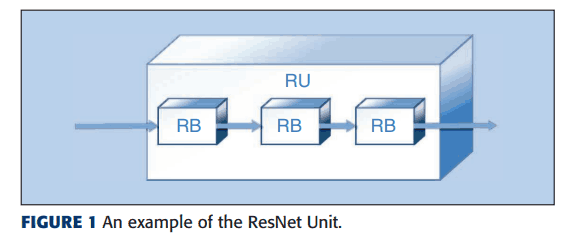In this research, we use such a unit as a basic unit to represent the architecture of CNN. There are three types of units as follows

1. ResNet Unit (RU)
2. DenseNet Unit (DU)
3. Pooling Unit (PU)

#### ResNet Unit (RU)

The number of ResNet blocks (RBs) is determined using random numbers. Each RB contains at least one 3*3 convolutional kernel. Such RBs are connected and grouped as shown in Figure 1 and are called ResNet Unit.

#### DenseNet Unit (DU)

The number of DenseNet blocks (DB) is determined using random numbers. Each DB contains at least one 3*3 convolutional kernel. The difference between ResNet Unit and DenseNet Unit is that ResNet Unit has skip connections while DU does not.

#### Pooling Unit (PU)

Contains one pooling layer of either type, the Average pooling layer or the Max pooling layer.

#### Examples of genes

Sequences of the above basic units (units) are treated as individuals as follows and genetic algorithms are executed.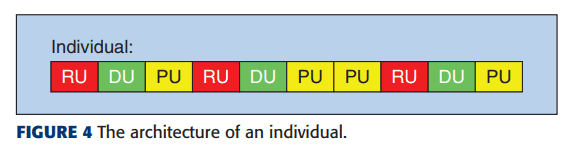### Initializing the first generation

The genes shown in the figure above are initially initialized using random numbers. A random number determines the length of each individual, and where each unit is placed is also determined using another random number. However, we prohibit PUs from coming first, because if individuals start with a PU, they will suddenly drop much of the information in the input. Also, if the PUs keeps coming, the output size will be 1*1 at that point, so there is a threshold for the number of times they can keep coming.

### Evaluation method of each individual

In the proposed method, each individual is decoded into a CNN architecture, which is trained and tested with training and validation data. The test score is used as the degree of adaptation of that individual.

### crossing methods

In the proposed method, a binary strategy is used to select the parents. In this method, two individuals are first randomly selected from the population, and then the individual with the highest fitness is selected as the parent, and mating is performed. To increase the flexibility of each individual, variable-length encoding is used instead of fixed-length encoding. This means that the depth of the CNN architecture is variable. In this case, the crossover is done using a single-point crossover.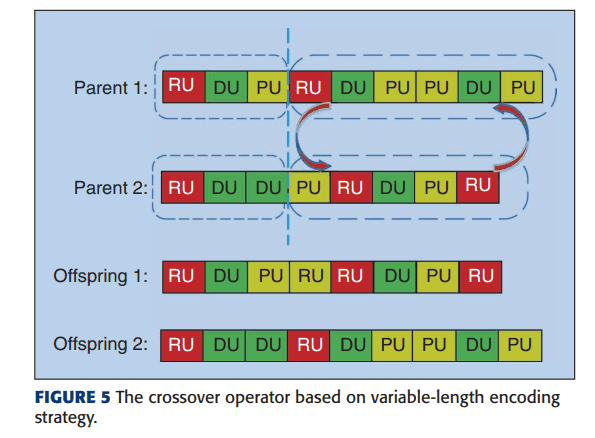After crossing, adjust the number of input channels and change the unit number.

### Method of Mutation (Self-Adaptive Mechanism)

In the proposed method, we add a twist to the mutation part of the general genetic algorithm. This section describes the innovations.

Three types of mutations are used: addition, deletion, and replacement, and any of these three are performed as a mutation operation. Addition refers to adding a unit at a selected position of an individual, deletion refers to removing a unit at a specified position of an individual, and replacement refers to randomly replacing a unit at a selected position of an individual.

The proposed method selects a good one among these three mutation operations and executes it. Specifically, the mutation operation is initially selected among the three with equal probability, but the selection probability of the mutation operation in the next generation is changed using the ratio of the performance of the individual after each mutation that exceeds that of the parent. The probability is changed so that the operation that outperforms the parent is more likely to be selected.

### Choosing the Next Generation

In the proposed method, the selection method for the next generation is also devised for the general genetic algorithm. Generally, when using a binary strategy, two randomly selected individuals are pitted against each other, and the one with the higher performance is left for the next generation. In this way, there is a problem that when two individuals with high performance are compared, the one with high performance cannot remain in the next generation, or when two individuals with low performance are compared, the one with low performance remains in the next generation.

To solve this problem, the proposed method generates a random number of individuals and leaves the top adapted individuals for the next generation. After that, for the remaining individuals, a dichotomous strategy is used to determine which individuals to leave for the next generation. By doing so, the problem of dichotomy is solved.

## experiment

In this paper, we evaluate the performance of the algorithm using the classification error rate, the number of parameters (i.e., the size of the model), and the computational resources (GPUs/days) to run the algorithm.

### Performance of models explored by the proposed method

The results of comparing each method with CIFAR10 and CIFAR100 are shown below.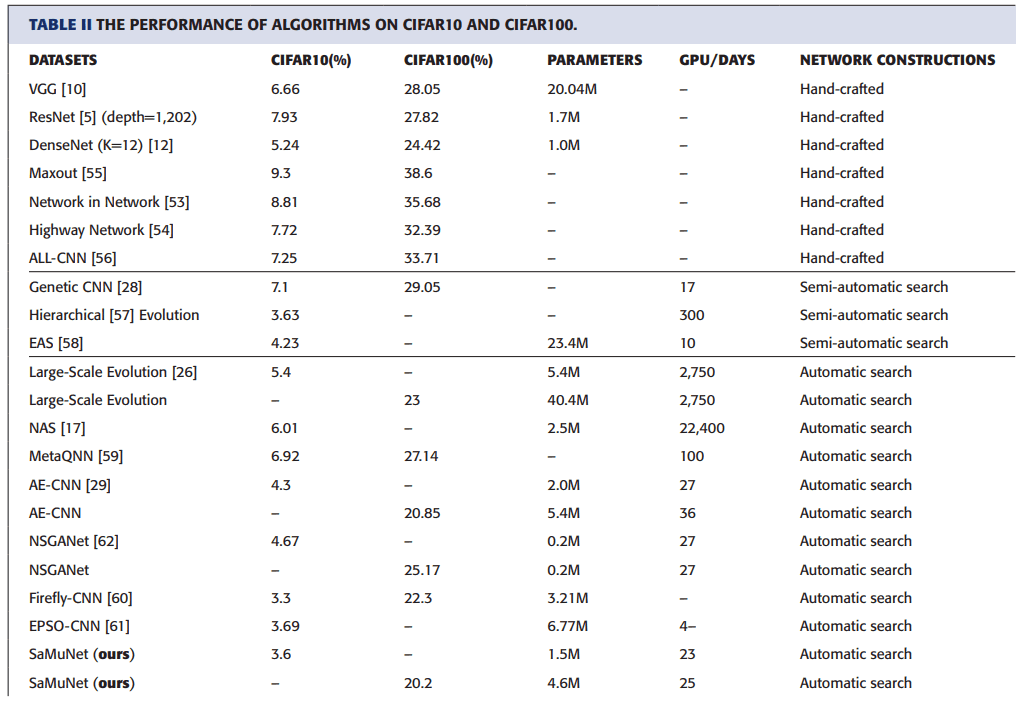Columns 2 and 3 show the error rates for CIFAR10 and CIFAR100, column 4 shows the final model size, column 5 shows the GPU/ days, and the sixth column shows how the model was constructed.

The table shows that compared to pure hand-made models including ResNet and DenseNet, the classification accuracy of SaMuNet in CIFAR10 achieves the second lowest error rate, which is slightly higher than that of Firefly-CNN. Furthermore, we can see that the model size is much smaller than Firefly-CNN at that time, and the GPU/days is also smaller than other automatic search algorithms.

### Whether the proposed method is effective or not

In the proposed method, we modified the general genetic algorithm with a change in the mutation-selection method and a change in the selection method for the next generation. Here we show the results of the verification of the effectiveness of the method.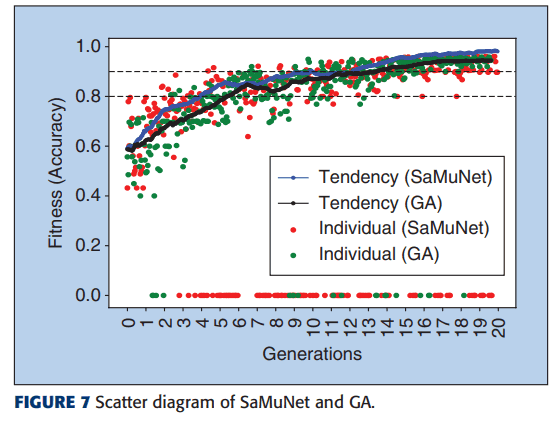This figure plots the relationship between the accuracy of the model of the search result and the generation when the general genetic algorithm (GA) and the proposed method are used to search. This figure shows that the proposed method can search models with higher performance in the same generation than the general genetic algorithm.

## summary

In this paper, the authors aimed to reduce the computational resources required for NAS and succeeded in searching models with relatively high classification accuracy while reducing the required computational resources by using a genetic algorithm and making the search space smaller by making it block-based. The authors mentioned at the end of the paper that they are aiming to further reduce the computational resources, so it will be very interesting to see how much they can reduce the resources in the future.

If you have any suggestions for improvement of the content of the article,